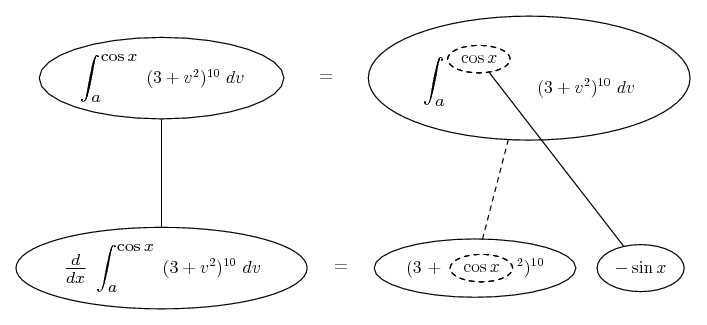# Thread: Find derivative using Part 1 of the Fundamental Theorem of Calculus?

1. ## Find derivative using Part 1 of the Fundamental Theorem of Calculus?

I never seen this problem before! I tried expanding and doing all that stuff but I got the wrong answer!

The function is (3+v^2)^10 with limits of integration [sin(x), cos(x)]2. Fundamental theorem of calculus - Wikipedia, the free encyclopedia

Just in case a picture helps...... differentiating downwards with respect to x (on the left) or with respect to the dashed balloon (right), the latter referring to chain rule, as below.

You might like to use properties of definite integrals to split the integral around a constant a.

For the half from a up to cos x...... where (key in spoiler) ...

Spoiler:... is the chain rule. Straight continuous lines differentiate downwards (integrate up) with respect to the main variable (in this case ), and the straight dashed line similarly but with respect to the dashed balloon expression (the inner function of the composite which is subject to the chain rule).

So you have half of the derivative you seek, on the bottom row, and you'll want to subtract a similar result got from applying the same process but with sin in the dashed balloon.
_________________________________________

Don't integrate - balloontegrate!

Balloon Calculus; standard integrals, derivatives and methods

Balloon Calculus Drawing with LaTeX and Asymptote!

#### Search Tags

calculus, derivative, fundamental, theorem